Symbol
Problem$x+10=-3x+38$ Show Your Work $2$ Solve the Equation: $x-6=5x-2$ Show Your Work
Other
Solution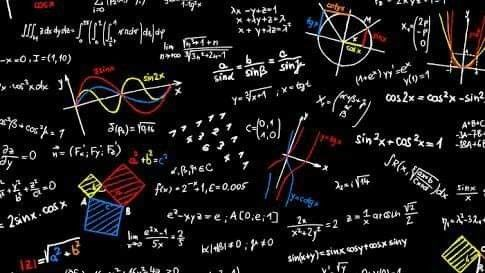Qanda teacher - StudyPoint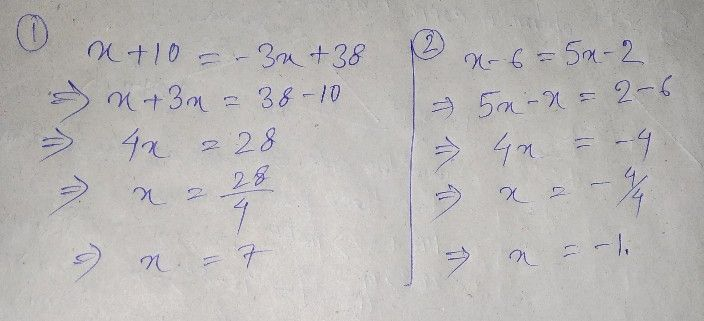Student
I’m sorry I don’t understand your handwritingQanda teacher - StudyPoint
waitStudent
OkQanda teacher - StudyPoint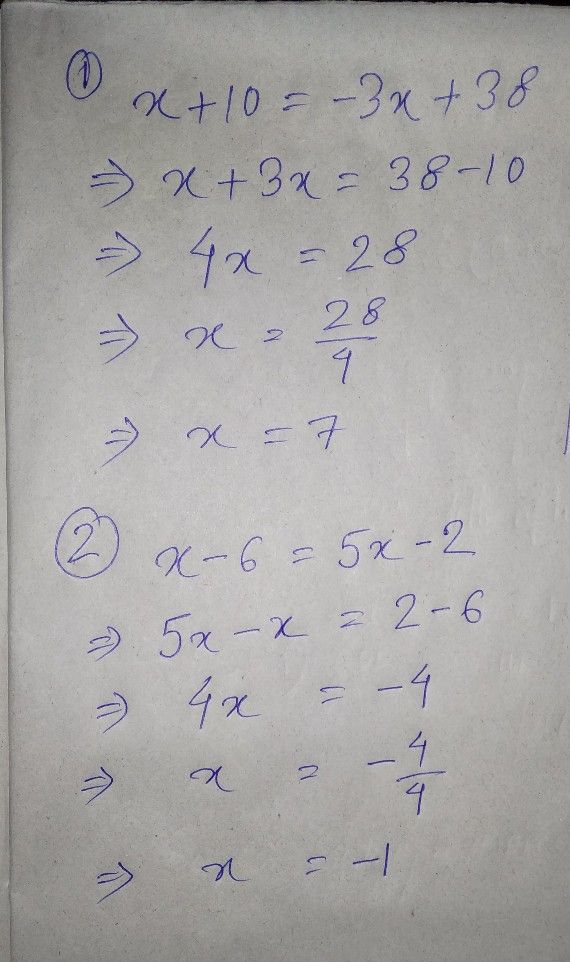Are you understand?Student
Yep thank u
Can u help wiht a couple more?Qanda teacher - StudyPoint
If you will give thank you gift and 5 start ? rating I must do itStudent
YessQanda teacher - StudyPoint
okStudent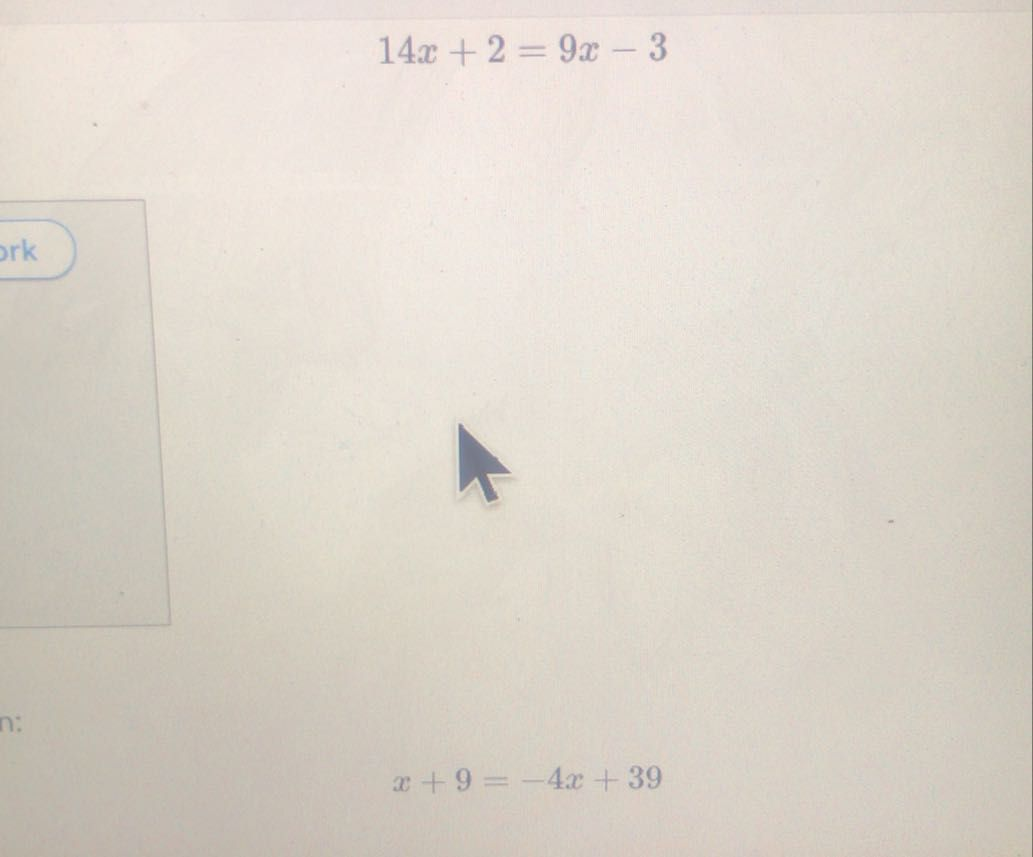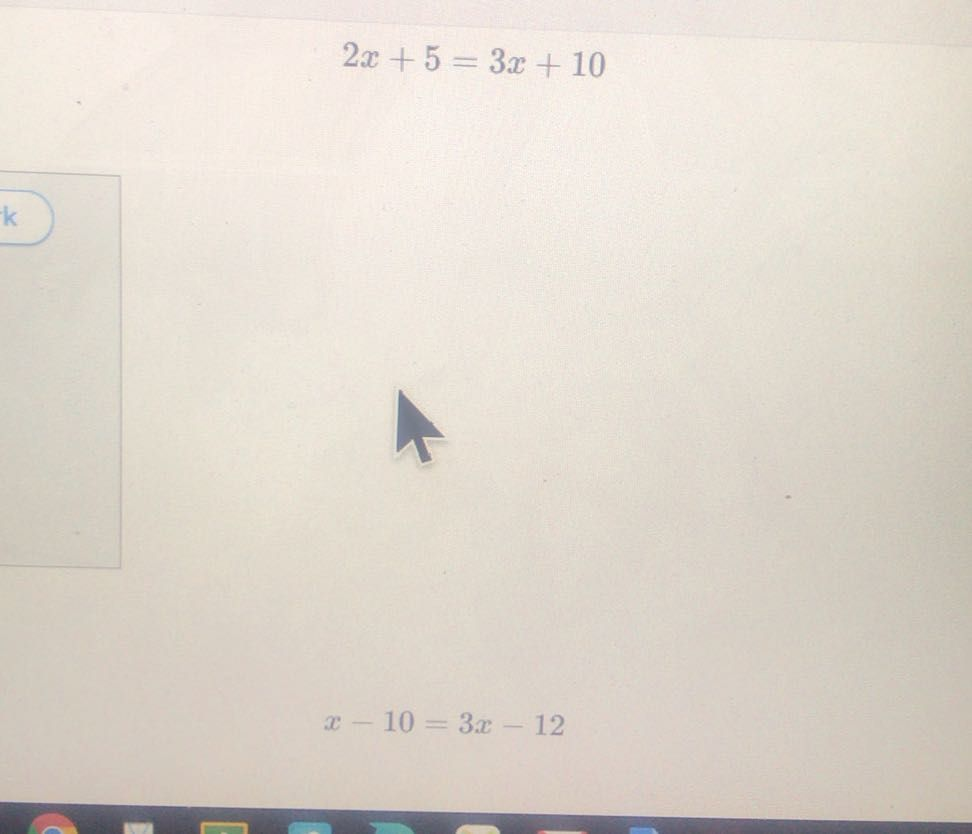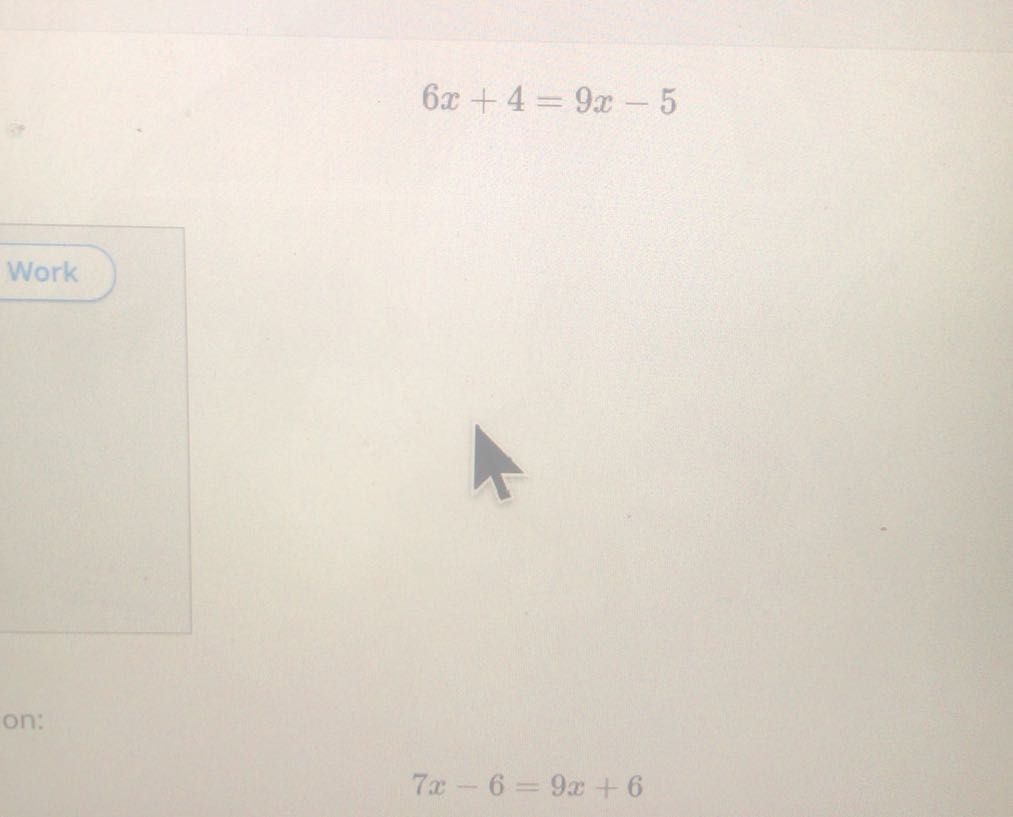Qanda teacher - StudyPoint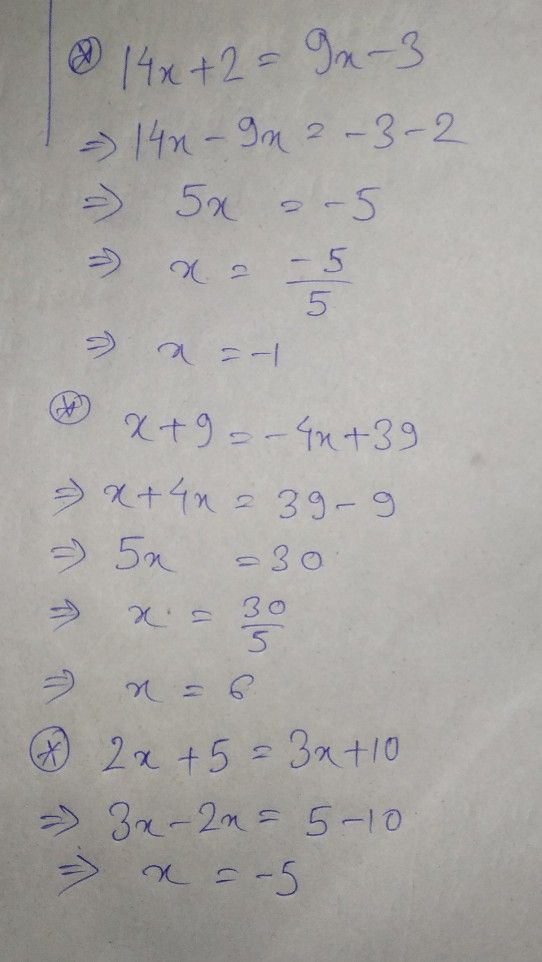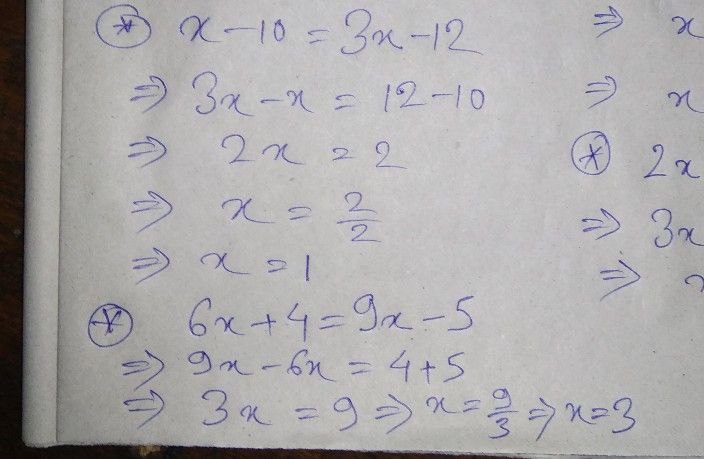Student
Thank you very muchQanda teacher - StudyPoint
welcome my dear student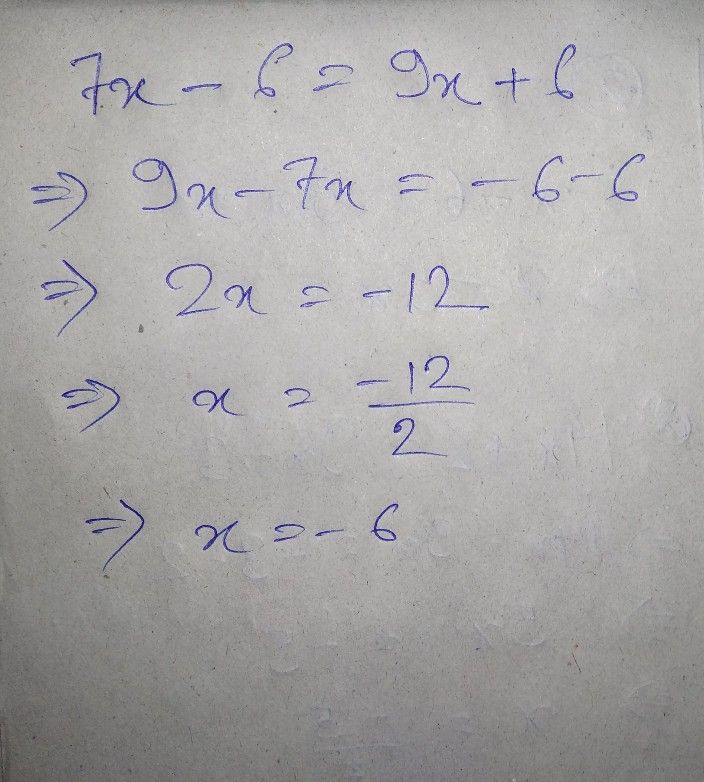Please evaluate the answer. If you are satisfied give 5 star rating . You give thank you gift